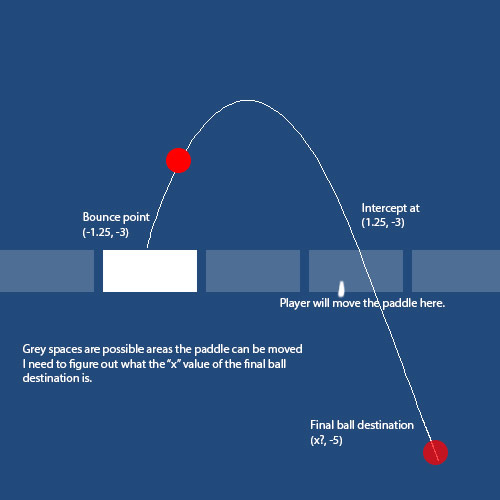# Creating a bouncing game without using physics - Vector3 math problem,

So I apologize if I over-explain this.

I’m creating a bouncing without using gravity or physics materials. Right now there are five possible spots on the x-axis where a ball is able to be (on the x-axis the spaces are -2.5, -1.25, 0, 1.25, 2.5) the ball bounces up in the y-axis and is unaffected by z.

After an initial bounce, the ball is able to go to any of the 5 spaces outlined on the x-axis. The balls will travel in a curve and land on one of the 4 other possible spaces. It’s also set up to go down on the y-axis to -5 (the paddles that intercept the ball are laid out on -3 on the y-axis) so the objective of the game is to move the paddle to one of 5 spots to intercept the ball. For an example of this is, when a ball hits a paddle in one of the spots, it will travel from (-2.5, -3, 0) to (1.25, -5, 0)

However, because I have the balls going all the way down to -5 on the y-axis the spots where the paddle intercepts the ball are not in the middle of one of the points on the x-axis I outlined earlier (-2.5, -1.25 etc.), They end up being either short or over-extended where they should be because they need to travel the extra 2 to reach -5.

Setting the y value on the bounced position from -5 to -3 or so fixes the problem but then the animation ends and the ball doesn’t descend further. The solution I’m trying to figure out is instead of bouncing it on the x-axis on one of the bounce positions I outlined earlier (-2.5, 1.25 etc.), instead of aiming for one of the x-axis values directly, plot an imaginary point at -5 where the paddle space would intercept the ball. However, I don’t have the foggiest on the math I would need to calculate the x value of that imaginary point.

This is the code I’m using to plot the point currently

``````endPos = new Vector3 ((paddleSpaces [nextSpace].GetComponent<Transform> ().position.x), -5f, 0f);
``````

Where paddleSpaces is an array of GameObjects containing the five spaces, nextSpace is a random number between 0 and 4.

And this is what I’m using to calculate the curve animation

``````		while(Time.time < timeStamp + (bounceTime/gc.speedCo)){

Vector3 currentPos = Vector3.Lerp(startPos, endPos, (Time.time - timeStamp)/(bounceTime/gc.speedCo));

currentPos.x += bending.x * Mathf.Sin (Mathf.Clamp01 ((Time.time - timeStamp) / (bounceTime/gc.speedCo)) * Mathf.PI);
currentPos.y += bending.y * Mathf.Sin (Mathf.Clamp01 ((Time.time - timeStamp) / (bounceTime/gc.speedCo)) * Mathf.PI);
currentPos.z += bending.z * Mathf.Sin (Mathf.Clamp01 ((Time.time - timeStamp) / (bounceTime/gc.speedCo)) * Mathf.PI);

transform.position = currentPos;

if (bounced) {
yield break;
}

yield return null;
}
``````

Please, any help that you guys can offer me in this would be greatly appreciated. I confess I’ve been stuck on this problem for a couple days and my different attempts to solve it have not gotten me very far.

Thank you.
,

I added a visual representation of what I’m trying to accomplish. So the five spaces are being outlined as the white spaces (currently the paddle is resting on (-1.25, -3). The user is able to move to any of these spaces by touch. The paddle has successfully bounced the ball and now the ball is headed towards space (1.25, -3), this is selected at random, it could be any of the spaces.The ball must go down to -5 (to be considered a loss) and it’s the player’s job to intercept the ball at the paddle spot to keep the game in play before that. Once the ball gets to -5 on the y-axis, it’s considered a miss and the game is over.

So I know that on the path of this curve the ball will make, it must pass through (1.25, -3) to land smack dab in the middle of the paddle space for the paddle to intercept it. What I’m trying to figure out is what the x value of the final ball destination must be to accomplish that. As I said before the ball must get down to -5, but what’s the x-value going to be?

Instead of Lerping to the end position and stopping, allow the ball to pass through endPos and keep going. The loop would need to stop when y is less than -5 (or when there is a paddle bounce, but it looks like you handle that case already.)

To make the loop work this way you’d need to change a few things.

1. Replace while condition

while (Time.time < timeStamp + (bounceTime/gc.speedCo)){
with something like

while (transform.position.y >= -5.0f) {

2. Replace Lerp with LerpUnclamped, to allow t to go past 1.

3. I’m pretty sure you will need to remove the calls to Mathf.Clamp01. I don’t know why they are needed, and will probably prevent the curve from being smooth.

4. (optional) The code will be more readable if you calculate (Time.time - timeStamp)/(bounceTime/gc.speedCo) once and store it in a variable, e.g.

float scaledElapsedTime = (Time.time - timeStamp)/(bounceTime/gc.speedCo);
Vector3 currentPos = Vector3.Lerp(startPos, endPos, scaledElapsedTime);
// etc

You will probably need other changes as well, but hopefully you understand the idea.